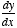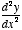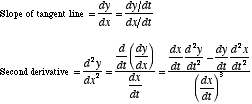# parametric derivative formulas

Parametric Derivative Formulas

The formulas for the first derivativeand second derivativeof a parametrically defined curve are given below.